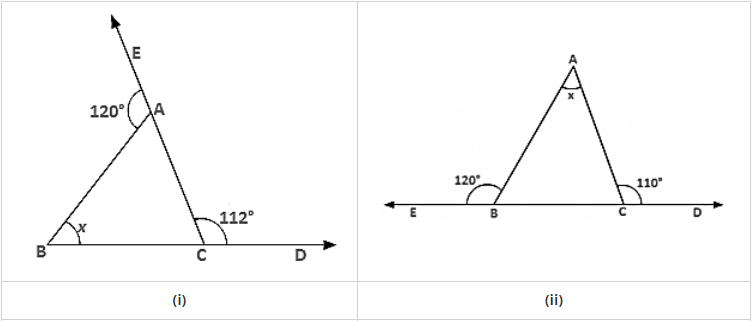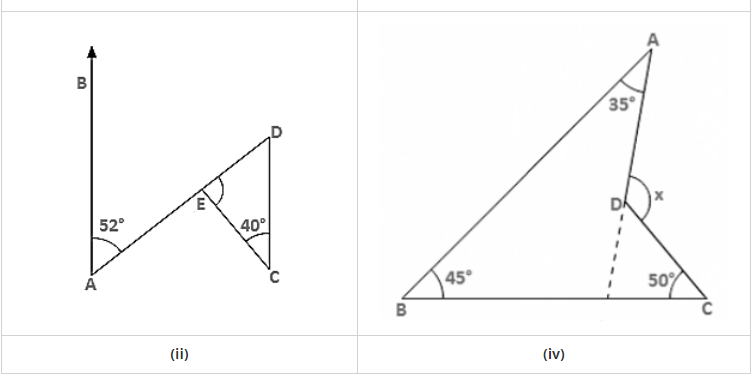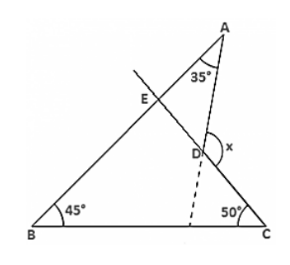# Compute the value of x in each of the following figures:

Question:

Compute the value of x in each of the following figures:Solution:

(i)  ∠BAC = 180° − 120° = 60° [Linear pair]

∠ACB = 180° − 112° = 68° [Linear pair]

∴ x = 180° − ∠BAC − ∠ACB

= 180° − 60° − 68° = 52° [Sum of all angles of a triangle]

(ii) ∠ABC = 180° − 120° = 60° [Linear pair]

∠ACB = 180° − 110° = 70° [Linear pair]

∴ e∠BAC = x

= 180° − ∠ABC − ∠ACB = 180° − 60° − 70° = 50° [Sum of all angles of a triangle](iii) ∠BAE = ∠EDC = 52° [Alternate angles]

∴ ∠DEC = x = 180° − 40° − ∠EDC

= 180° − 40° − 52°

= 180° − 92° = 88° [Sum of all angles of a triangle]

(iv) CD is produced to meet AB at E.

∠BEC = 180° − 45° − 50° = 85° [Sum of all angles of a triangle]

∠AEC = 180° − 85° = 95° [Linear pair]

∴ x = 95° + 35° = 130° [Exterior angle property].